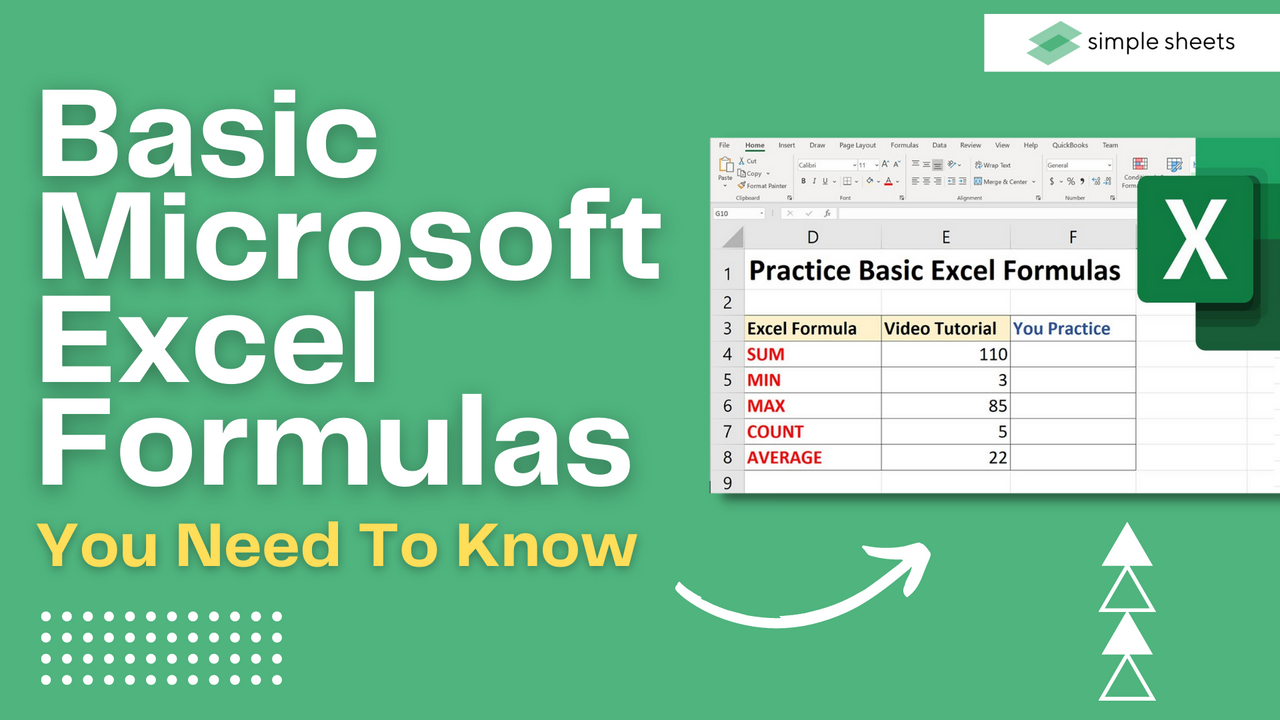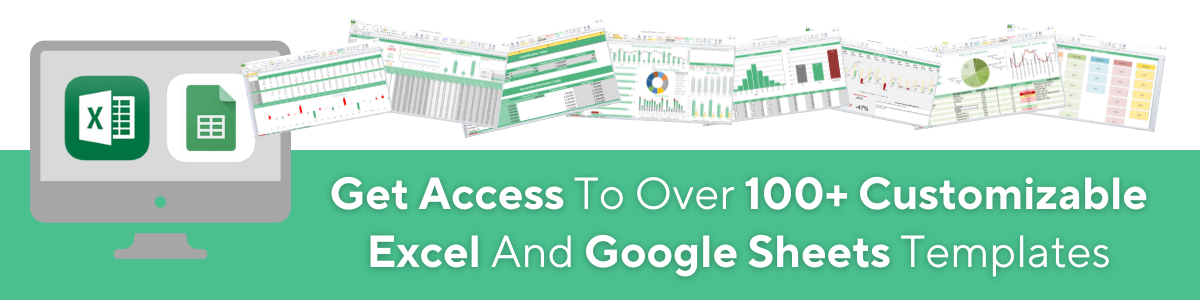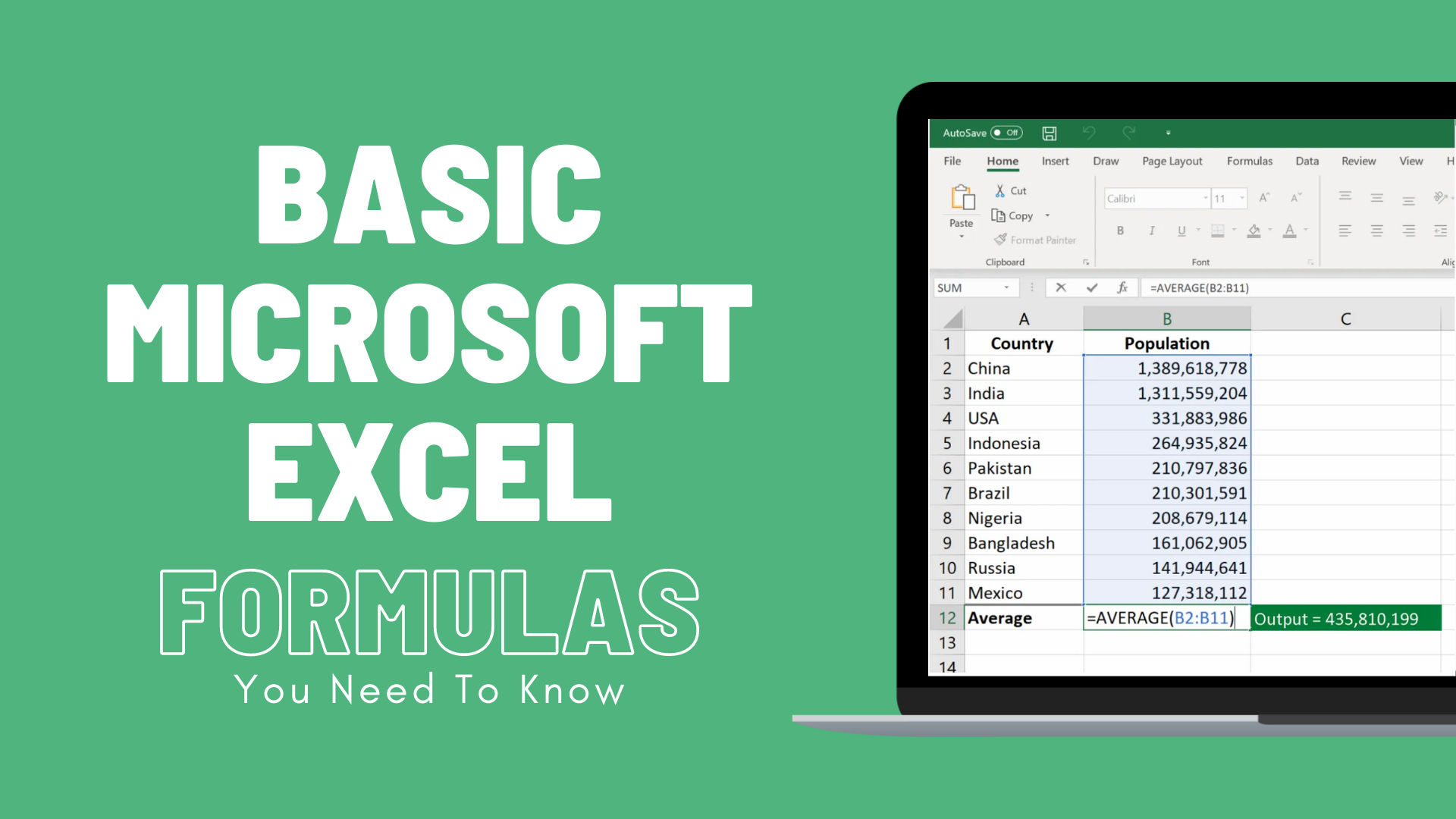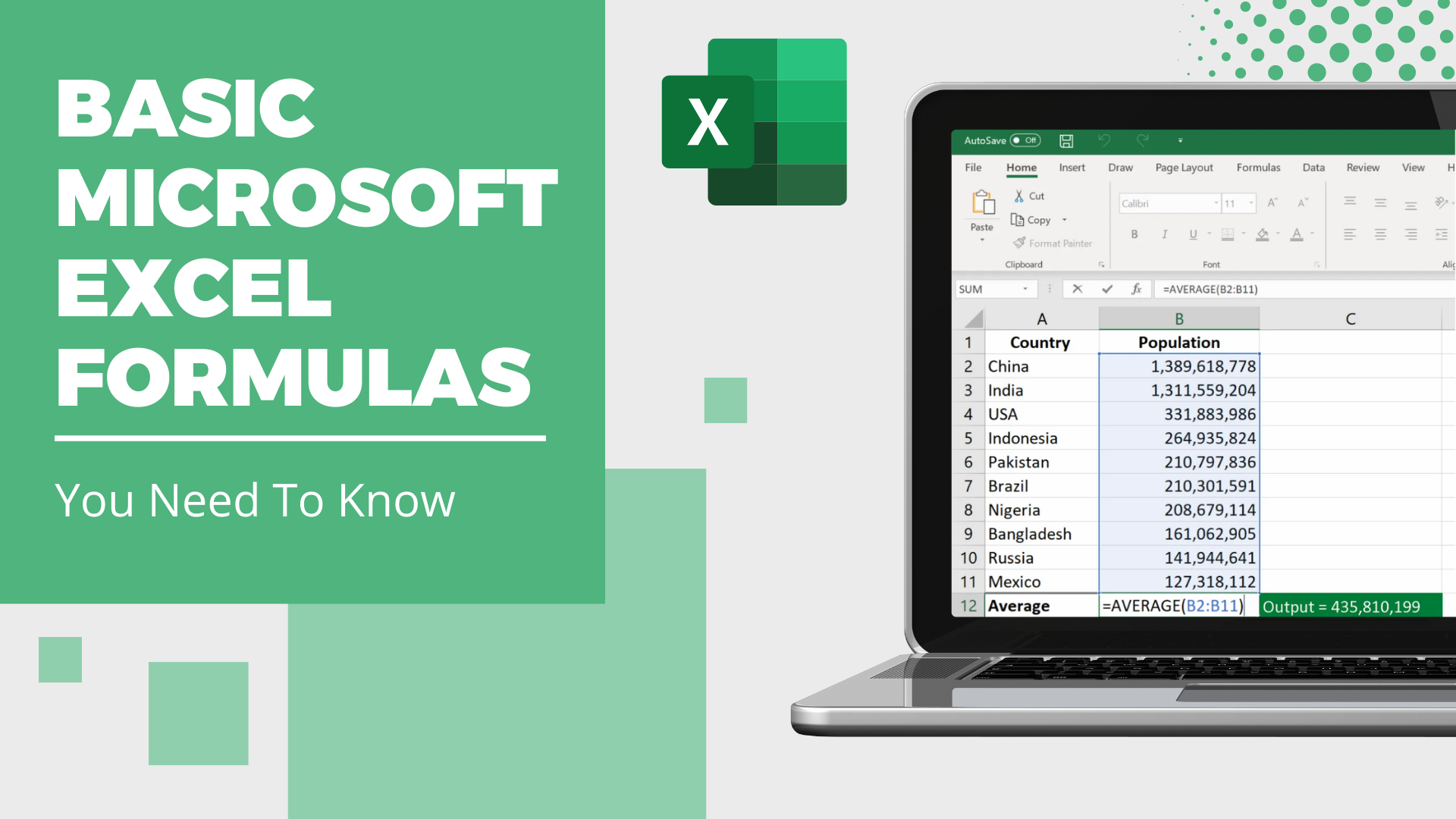# Basic Microsoft Excel Formulas You NEED to Know

Aug 10, 2022Getting comfortable with the basic formulas in Excel is essential for any beginner who wants to become proficient at using the software.

For many jobs, Microsoft Excel is viewed as the industry standard tool for data analysis and is preferred by countless businesses.## Basic Terms in Excel

Firstly, it is worth pointing out that there are two ways you can perform calculations in Microsoft Excel.

### Formulas.

In Excel, a formula can be defined as an expression that works on values in a cell or range of cells.

### Functions.

Functions, on the other hand, are predefined formulas within Excel. They take out all the time you would spend inputting formulas.## Five Time-saving Ways to Insert Data into Excel

When we analyze data in Excel, there are five different ways that many people choose to insert formulas. Every one of these choices has benefits and downsides. Before we look at the basic Excel formulas you can use, let's look at these methods so you can select the right one for your needs.

### Simple insertion: Typing a formula inside the cell.

Entering a formula into a cell or formula bar is the simplest and easiest way to insert a basic Excel formula. This process begins with an equal sign, then the name of your chosen function.

One of the benefits of working in Excel is that once you begin typing the name of your chosen function, a pop-up function hint will appear.

### Using Insert Function Option from Formulas Tab.

However, if you prefer complete control over your function insertion, the Excel Insert Function dialog box might be better for your needs.

Click on the Formulas tab and select Insert Function. A dialog box containing any function needed to execute your analysis will appear.

### Selecting a Formula from One of the Groups in the Formula Tab.

For any user that wants to get to their favorite functions quickly, the group within the Formula tab is a handy option.

Click on the Formulas tab and choose your preferred group. This action will show a sub-menu full of functions to pick from.

### Using AutoSum Option.

For simple everyday tasks, the AutoSum function is fantastic. Head to the Formulas tab and select the AutoSum option. Then select the caret to show other hidden formulas. You can also get to this option via the Home tab.

### Quick Insert: Use Recently Used Tabs.

If you notice that you use a particular formula often and re-typing it each time is taking a lot of time, then you can use the Recently Used option. Select the third menu option from the Formulas tab next to AutoSum.

## Basic Excel Formulas For Your Workflow

Now that you understand how to insert formulas and functions correctly, let's look at some basic Excel formulas and functions you can use to improve your spreadsheet.### SUM.

Firstly, the SUM function is the most important formula to learn in Excel. This function aggregates values from chosen columns or rows.

Here is the syntax:

=SUM(number1, [number2], …)

### AVERAGE.

The AVERAGE function allows you to find the average number in a list of data. It might be in the same cell as you estimated it to be, but to be sure, an AVERAGE function is an excellent tool.

Here is the syntax:

=AVERAGE(number1, [number2], …)

### COUNT.

The COUNT function allows you to count all the values in various cells containing numeric values.

Here is the syntax:

=COUNT(value1, [value2], …)### COUNTA.

Like the COUNT function we discussed, COUNTA counts only the cells in a chosen range. However, this function counts all cells, no matter their type. The COUNT function only counts numerical values, but COUNTA also counts times, dates, logical values, text, errors, and strings, which is why it is not the same formula.

Here is the syntax:

=COUNTA(value1, [value2], …)

### IF.

The IF function tends to sort data according to a given logic. One of the benefits of using the IF formula in Excel is that you can embed other formulas within it.

Here is the syntax:

=IF(logical test, [value_if_true], [value_if_false])

### TRIM.

Finally, the TRIM function is perfect for ensuring any Excel function you implement does not return errors because of extra spaces in the data.

It allows you to eliminate all of the empty spaces. Other Microsoft Excel functions can operate across multiple cells, but TRIM can only work in one cell. Therefore, it can be frustrating to use in some instances.

Here is the syntax:

=TRIM(text)

## Excel Formulas: Summary and Key Takeaways

Now you have all the information you need to thrive on the Excel program! For more free Excel advice and stunning pre-made templates that will add maximum value to your spreadsheets, check out the rest of the Simple Sheets website.

### Can you use an Excel Formula to Analyze Data?

Correct Excel formulas are the best way to study numerical values and understand data. Formulas allow you to study data from multiple cells, compare columns a and b, and in many other ways, you can utilize them.

### Is the SUM function easy to use in an Excel spreadsheet?

The SUM formula is one of the most specific functions you can use in Excel. Follow the steps below to find the insert function button for the SUM formula and quickly add up your cell values.

### What are the essential Excel formulas?

1. SUM

2. AVERAGE

3. COUNT

4. COUNTA

5. IF

6. TRIM## Related Articles to Excel Formulas and Functions:

Excel: Remove Trailing Spaces Quickly and Easily With These Simple Steps

Excel Repeat Last Action: What is it and How Does it Work?

SUM Index-Match: What is it, and How do I use it?

#### Want to Make Excel Work for You? Try out 5 Amazing Excel Templates & 5 Unique Lessons

We hate SPAM. We will never sell your information, for any reason.